### Bucket Sort Algorithm

Introduction:

Bucket Sort is a sorting technique that sorts the elements by first dividing the elements into several groups called buckets. The elements inside each bucket are sorted using any of the suitable sorting algorithms or recursively calling the same algorithm. Several buckets are created. Each bucket is filled with a specific range of elements. The elements inside the bucket are sorted using any other algorithm. Finally, the elements of the bucket are gathered to get the sorted array.

The process of bucket sort can be understood as a scatter-gather approach. The elements are first scattered into buckets then the elements of buckets are sorted. Finally, the elements are gathered in order.

Example: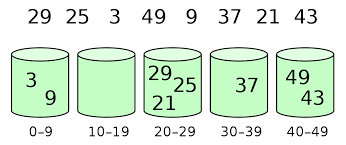Program to implement Bucket Sort in C++: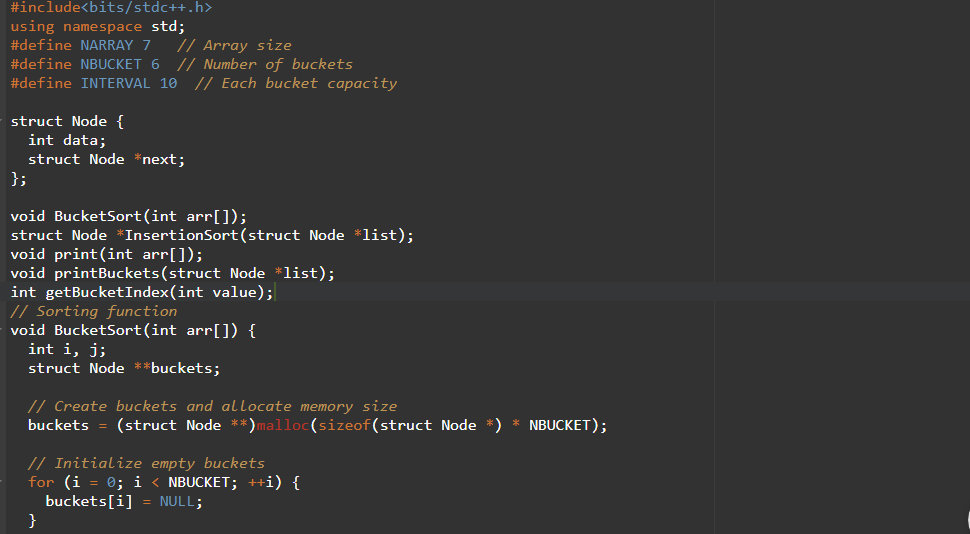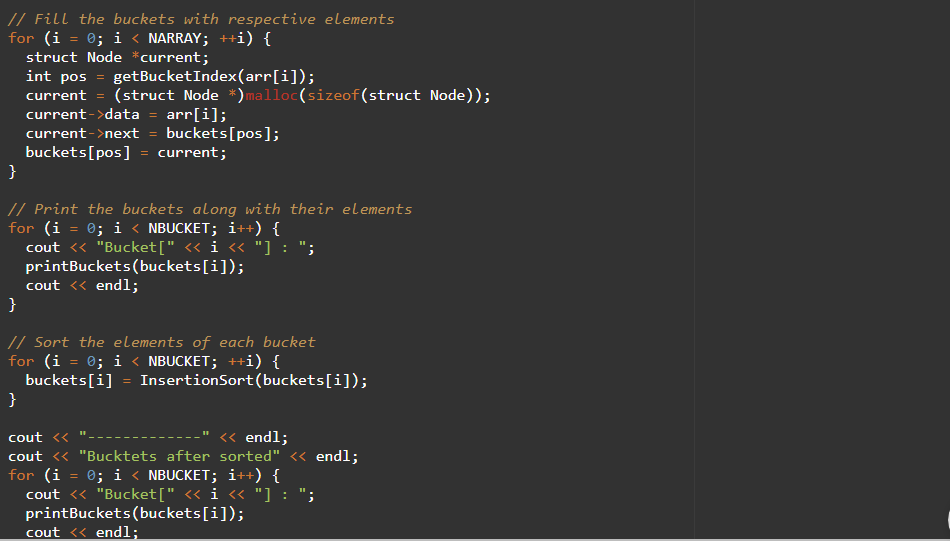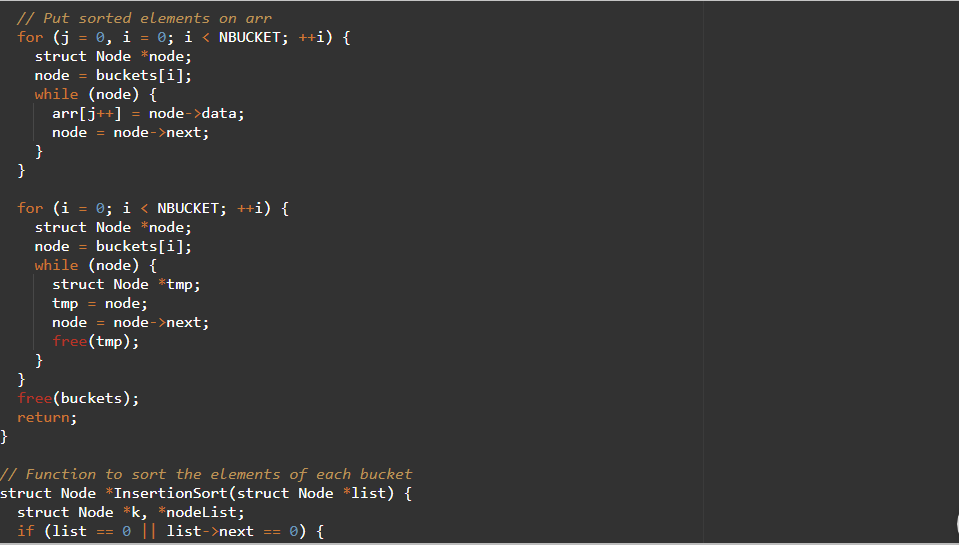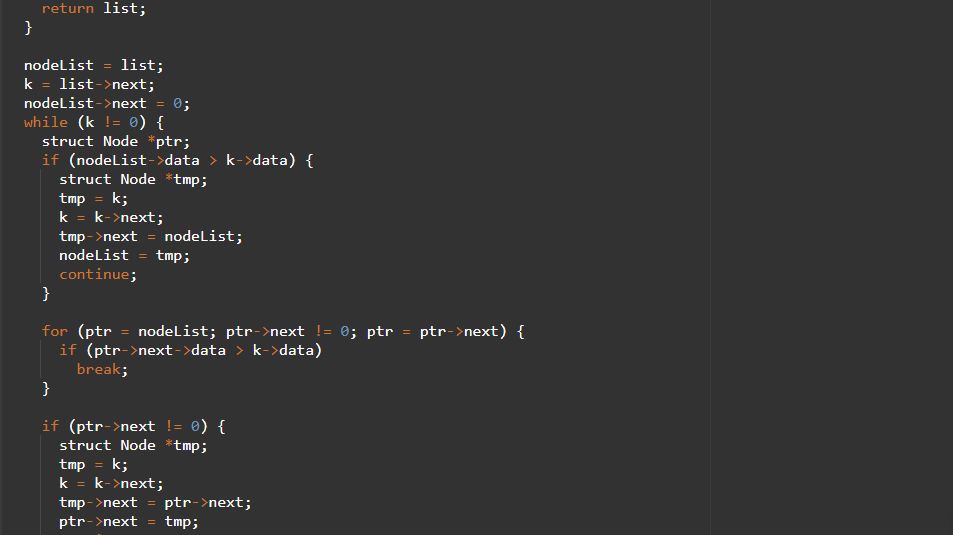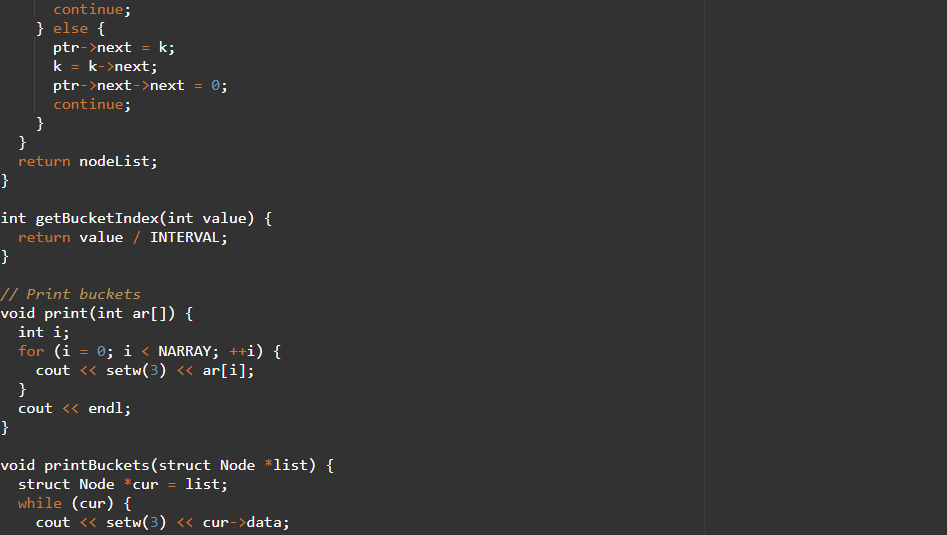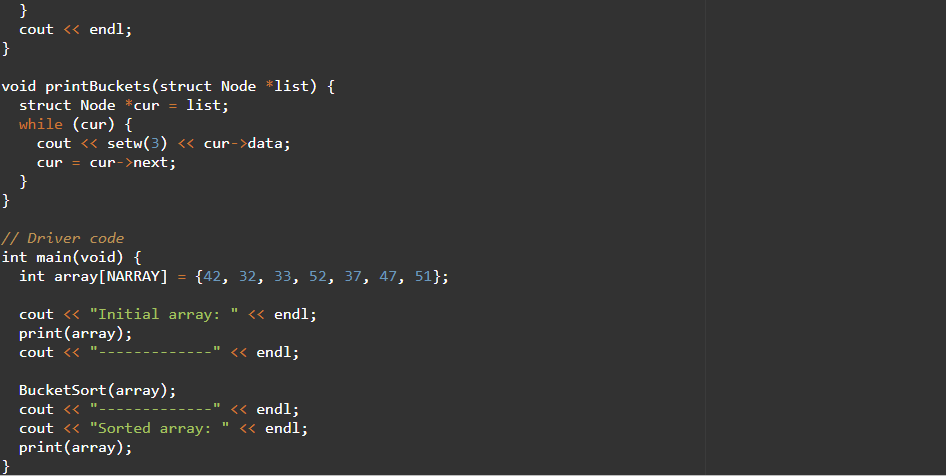OUTPUT: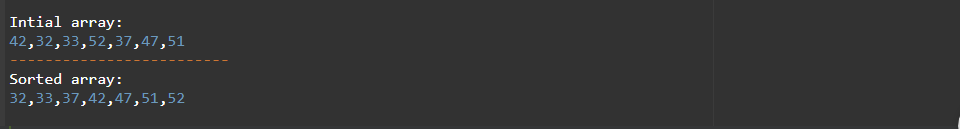## Bucket Sort Applications

Bucket sort is used when:

• input is uniformly distributed over a range.
• there are floating-point values